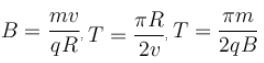Courses

# Magnetic Effect Of Current MSQ

## 10 Questions MCQ Test Basic Physics for IIT JAM | Magnetic Effect Of Current MSQ

Description
This mock test of Magnetic Effect Of Current MSQ for Physics helps you for every Physics entrance exam. This contains 10 Multiple Choice Questions for Physics Magnetic Effect Of Current MSQ (mcq) to study with solutions a complete question bank. The solved questions answers in this Magnetic Effect Of Current MSQ quiz give you a good mix of easy questions and tough questions. Physics students definitely take this Magnetic Effect Of Current MSQ exercise for a better result in the exam. You can find other Magnetic Effect Of Current MSQ extra questions, long questions & short questions for Physics on EduRev as well by searching above.
*Multiple options can be correct
QUESTION: 1

### A Positive point charge is moving in clockwise direction in a circle with constant speed. Consider the magnetic field produced by the charge at a point P (not centre of the circle) on the axis of the circle. Which of the following is incorrect.

Solution:

The point charge moves in circle as shown in figure. The magnetic field vectors at a point  P  on axis of circle are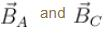at the instants the point charge is at A and C respectively as shown in the figure.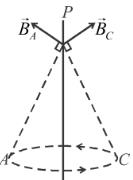Hence as the particles rotates in circle, only magnitude of magnetic field remains constant at the point on axis P but its direction changes.

The correct answers are: it is constant in direction only, it is constant in direction and magnitude both, it is not constant in magnitude and direction both.

*Multiple options can be correct
QUESTION: 2

### The negatively and uniformly charged nonconducting disc as shown in the figure is rotated clockwise with great angular speed. Which of the following is not the direction of the magnetic field at point A in the plane of the disc :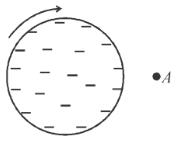Solution: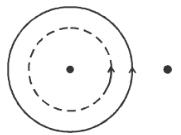Disc behaves like made up of coils arranged in a plane in which current is flowing in anti-clockwise direction.
Hence, the field at  A  is direction into the page.
The correct answers are: out of the page, up the page, down the page

*Multiple options can be correct
QUESTION: 3

### In a region magnetic field along x-axis changes with time according to the given graph. If time period, pitch and radius of helix path are T0, P0 and R respectively then which of the following is correct if the particle is projected at an angle θ0 with the positive x-axis in x-y plane.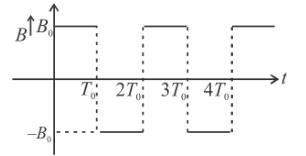Solution:

As the magnetic field is along the x-axis, the magnetic force will be along negative z-axis from t = 0 to t = T0 and along positive z-axis fromt = T0  to  t = 2T0
For    t = 0  to  t = T0

At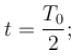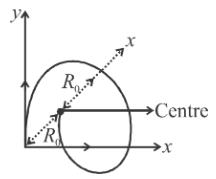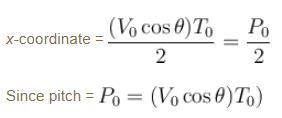y-coordinate = 0 (from figure)
and  z-coordinate = –2R0  (from figure)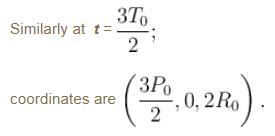The correct answers are: At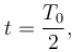co-ordinates of charge are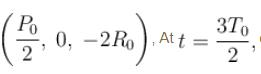co-ordinates of charge are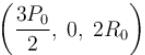Two extremes from x-axis are at a distance 2R0  from each other.

*Multiple options can be correct
QUESTION: 4

Three infinite current carrying conductors are placed as shown in figure. Two wires carry same current while current in third wire is unknown. The three wires do not intersect with each other and all of them are in the plane of paper. Which of the following is incorrect about a point ‘P’  which is also in the same plane :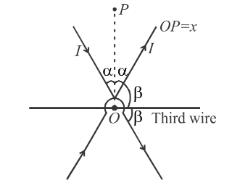Solution:

Magnetic field at  ‘P’  due to wires (1) and (2) is :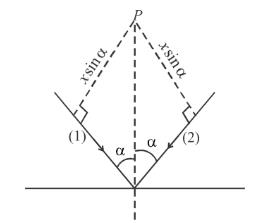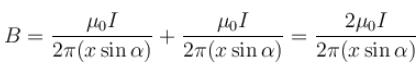(out of the paper)

The correct answers are: Magnetic field intensity at P  is zero for all values of  x., If the current in the third wire is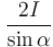(left to right) then magnetic field will be zero at P  for all values of x., If the current in the third wire is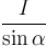(right to left) then magnetic field will be zero at  P  for all value of  x.

*Multiple options can be correct
QUESTION: 5

From a cylinder of radius R, a cylinder of radius R/2 is removed, as shown. Current flowing in the remaining cylinder is I. Magnetic field strength is :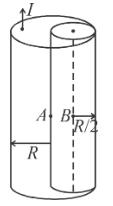Solution:

For cylinder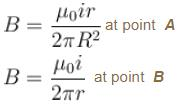We can consider the given cylinder as a combination of two cylinders. One of radius ‘R’  carrying current. I  in one direction and other of radius R/2 carrying current I/3 in both directions.The correct answers are: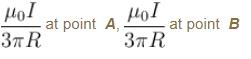*Multiple options can be correct
QUESTION: 6

A nonconducting disc having uniform positive charge Q, is rotating about its axis in anticlockwise direction with uniform angular velocity ω. The magnetic field at the center of the disc is.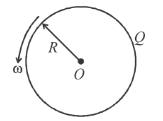Solution: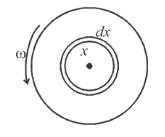Consider a ring of radius x and thickness dx.

Equivalent current in this ring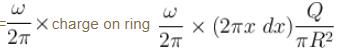dB (due to this ring)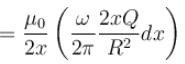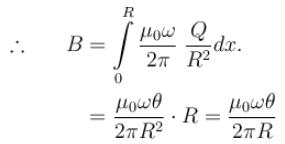The correct answers are: directed outward, having magnitude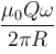*Multiple options can be correct
QUESTION: 7

A long thick conducting cylinder of radius ‘R’ carries a current uniformly distributed over its cross section.

Solution: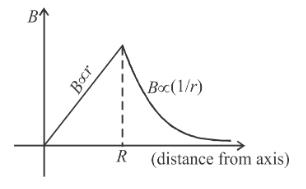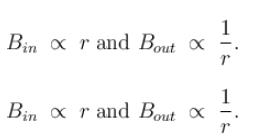Alterante solution
B (inside the conductor) ∝ r,  B(outside the conductor)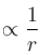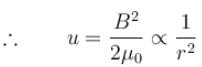The correct answers are: The magnetic field strength is maximum on the surface, The energy density of the magnetic field outside the conductor varies as inversely proportional to 1/r2, where ‘r’  is the distance from the axis.

*Multiple options can be correct
QUESTION: 8

A charge particle of charge q, mass m is moving with initial velocity v as shown in figure in a uniform magnetic field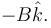Select the correct alternative/alternatives :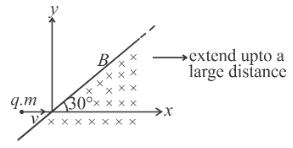Solution: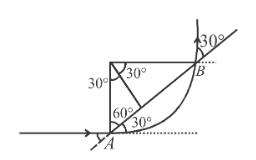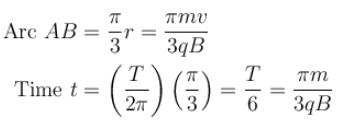The correct answers are: Velocity of particle when it comes out from magnetic field is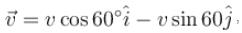Time for which the particle was in magnetic field is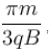Distance traveled in magnetic field is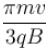*Multiple options can be correct
QUESTION: 9

If a charged particle of charge to mass ratio q/m = α is entering in a uniform magnetic field of strength B which is extended up to 4d as shown in figure at a speed v = 2αdB,  then which of the following is incorrect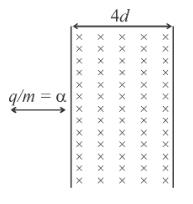Solution:

Electromagnetic force will provide the necessary centripetal force.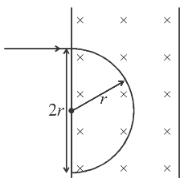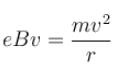⇒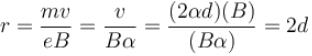i.e. the electron will move out after traveling on a semicircular path of radius r = 2d.

The correct answers are: angle subtended by charged particle at the centre of circular path is  2π, the time for which particle will be in the magnetic field is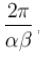the charged particle will subtended an angle of 90° at the centre of circular path

*Multiple options can be correct
QUESTION: 10

A particle of charge ‘q’ and mass m’ enters normally (at point P) in a region of magnetic field with speed v. It comes out normally from Q after time T as shown in figure. The magnetic field B is present only in the region of radius R and is constant and uniform. Initial and final velocities are along radial direction and they are perpendicular to each other. For this to happen, which of the following expression(s) is/are correct :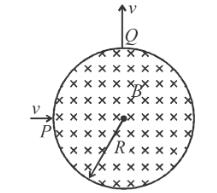Solution:

The particle will move along an arc which is part of a circle of radius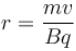From the figure we can see  r = R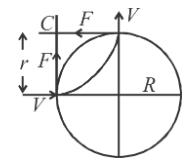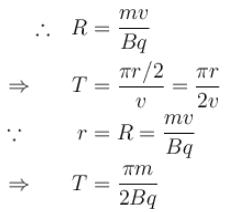The correct answers are: